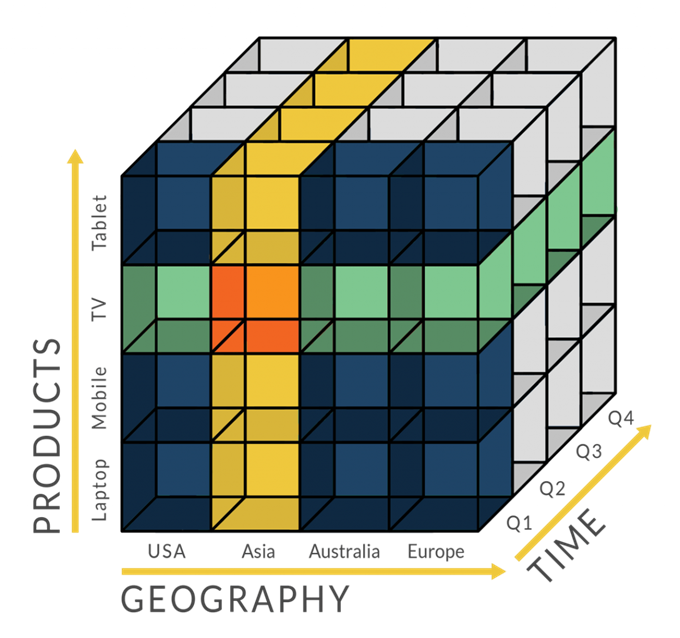# 使用Python将图片转化为ASCII字符图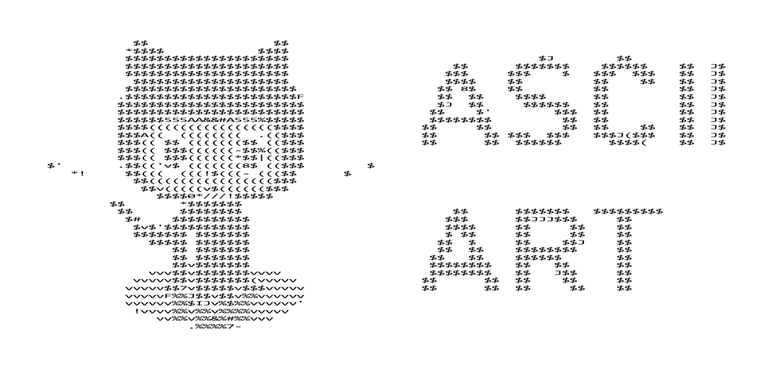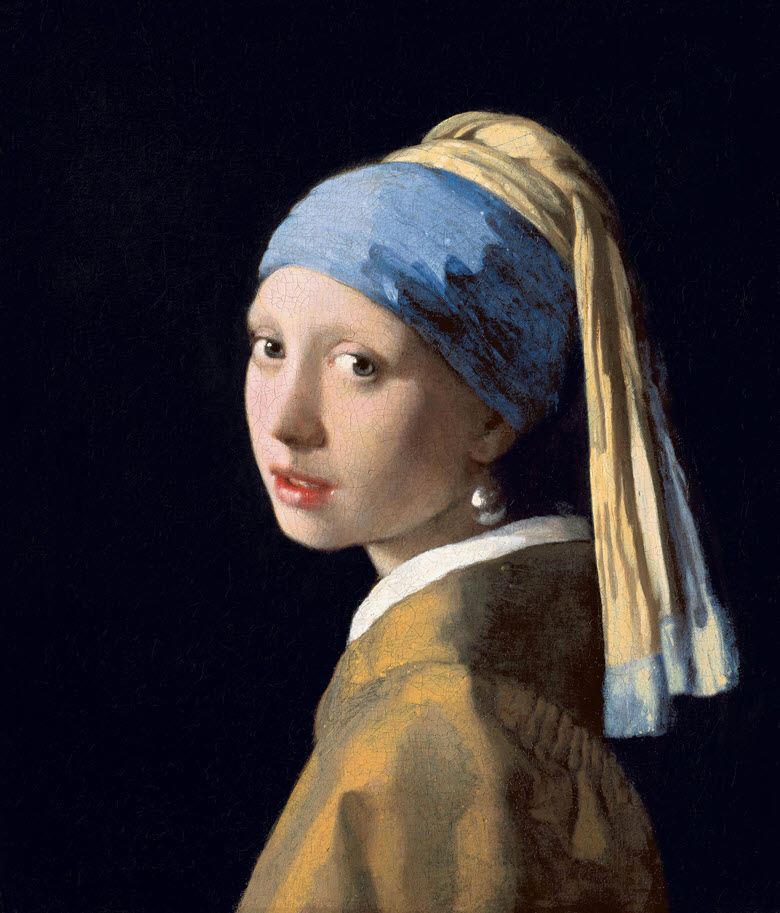• 彩色图像中的单个像素可以表示成一个三元组，其中每个元素取值都介于0和255之间，分别表示红色，绿色和蓝色的值。这些值越高，表示相应通道的亮度越大。例如，白色像素的三元组表示为(255,255,2555)。
• 灰度图又称灰阶图。 把白色与黑色之间按对数关系分为若干等级，称为灰度。 灰度分为256阶。 用灰度表示的图像称作灰度图。255代表全白，0表示全黑。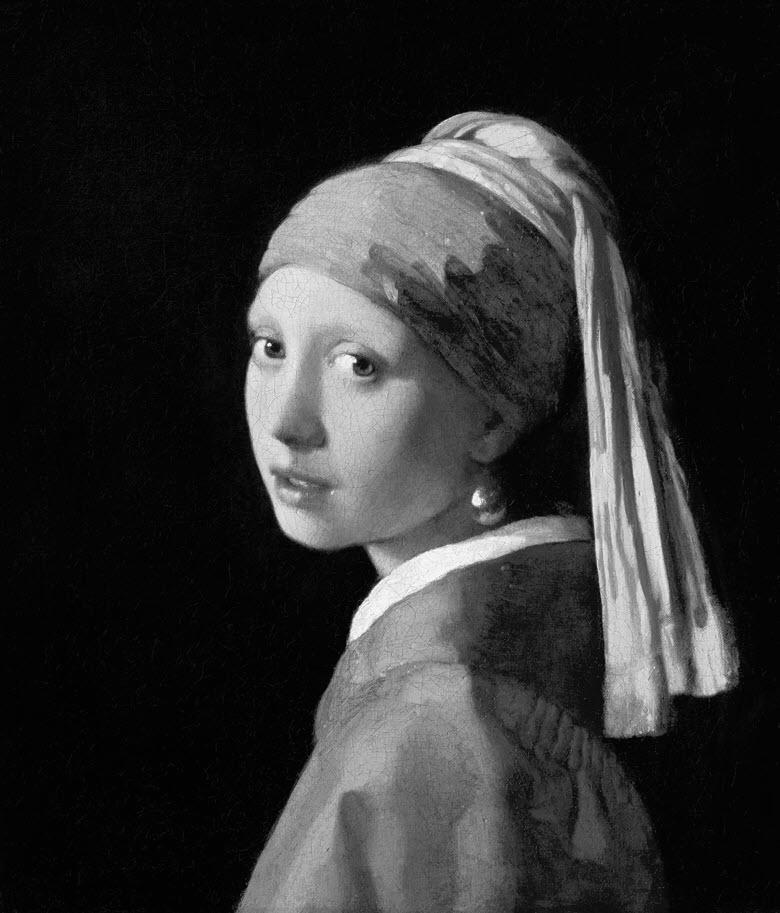CHARACTERS = (' ', '.', '°', 'o', 'O', '*', '#', '@')

gscale1 = "\$@B%8&WM#*oahkbdpqwmZO0QLCJUYXzcvunxrjft/\|()1{}[]?-_+~i!lI;:,\"^". "    #70 levels of gray
gscale2 = "@%#*+=-:. "         #10 levels of gray

ASCII_CHARS = "戴珠珍环的耳女少"

from PIL import Image

# ASCII_CHARS = "@%#*+=-:. "
ASCII_CHARS = "戴珠珍环的耳女少"

def img2ascii(image_path, ascii_path, image_width=100):
im = Image.open(image_path).convert('L')
im.save('Gray-Scale-Image.jpg')
width, height = im.size
image_height = image_width * height // width
im = im.resize((image_width, image_height))
im.show()
pixels = im.getdata()
ascii_str = ""
for pixel in pixels:
ascii_str += ASCII_CHARS[len(ASCII_CHARS) * pixel // 256]
ascii_str_len = len(ascii_str)

ascii_img = ""
for i in range(0, ascii_str_len, image_width):
ascii_img += ascii_str[i:i + image_width] + "\n"

with open(ascii_path, "w") as f:
f.write(ascii_img)

if __name__ == "__main__":
image_path = 'Meisje_met_de_parel.jpg'
ascii_path = 'ascii_image.txt'
image_width = 256
img2ascii(image_path, ascii_path, image_width)`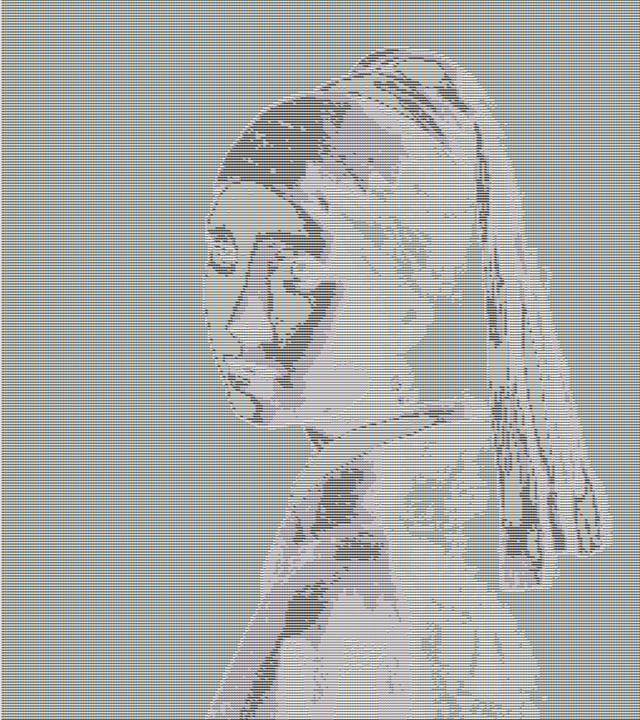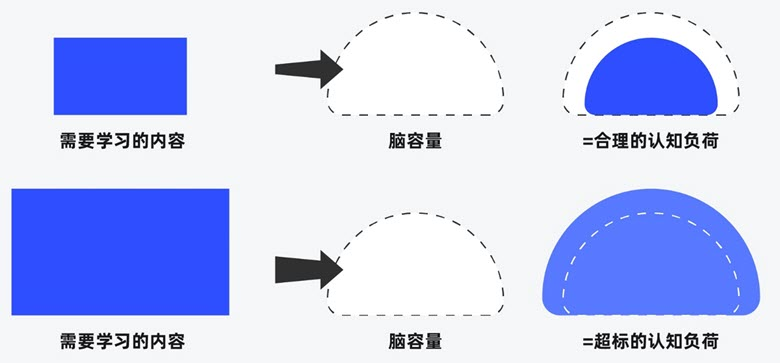##### 界面设计和游戏设计中的认知负荷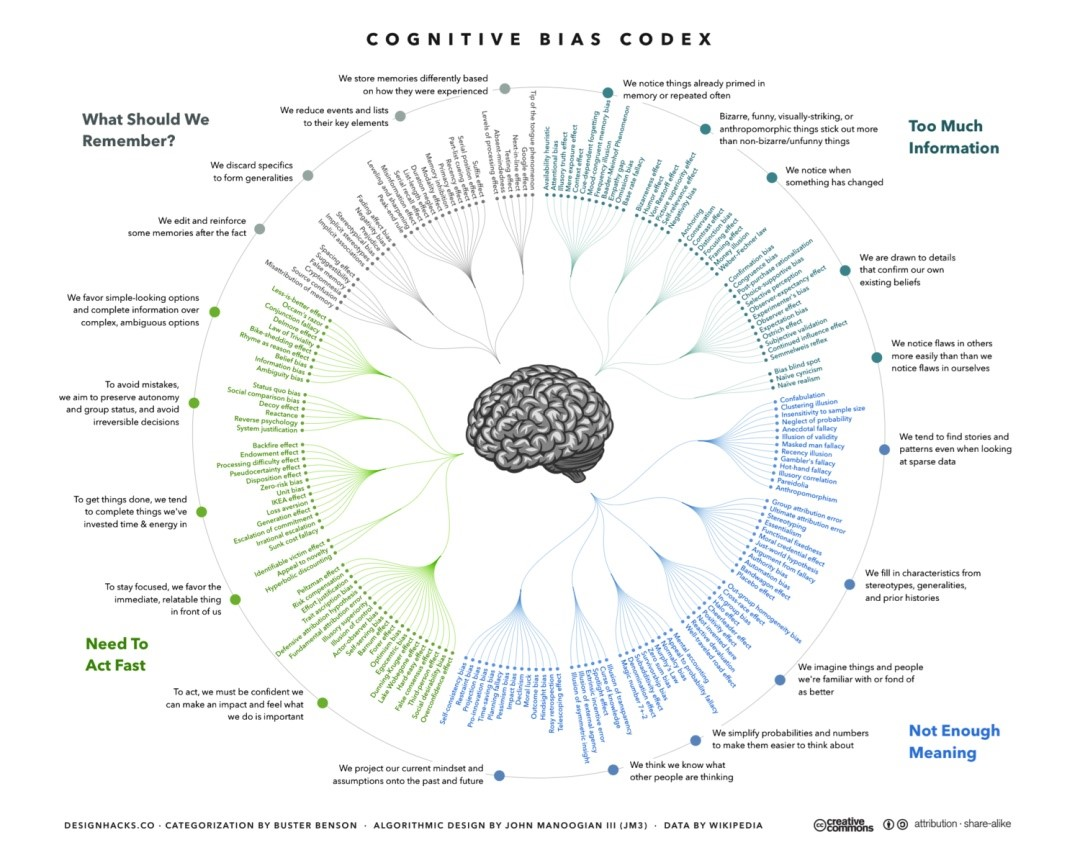##### 理解认知偏差